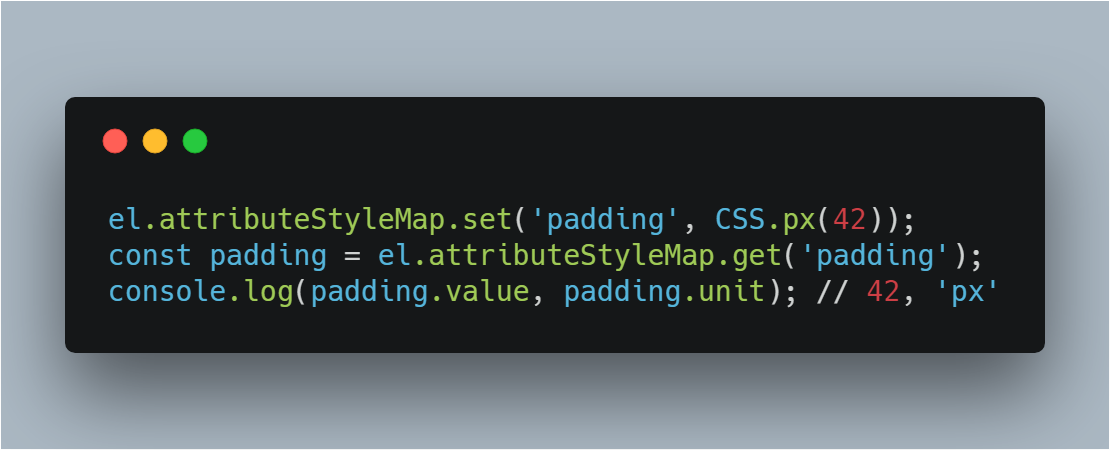## 0. 前言

``````el.attributeStyleMap.set('padding', CSS.px(42));
``````

## 1.1 旧的 CSSOM

``````// Element styles.
el.style.opacity = 0.3;
typeof el.style.opacity === 'string' // Ugh. A string!?

// Stylesheet rules.
document.styleSheets.cssRules.style.opacity = 0.3;
``````

## 1.2 新的 CSS Typed OM

``````// Element styles.
el.attributeStyleMap.set('opacity', 0.3);
typeof el.attributeStyleMap.get('opacity').value === 'number' // Yay, a number!

// Stylesheet rules.
const stylesheet = document.styleSheets;
stylesheet.cssRules.styleMap.set('background', 'blue');
``````

``````// All 3 of these are equivalent:
el.attributeStyleMap.set('opacity', 0.3);
el.attributeStyleMap.set('opacity', '0.3');
el.attributeStyleMap.set('opacity', CSS.number(0.3)); // see next section
// el.attributeStyleMap.get('opacity').value === 0.3

// StylePropertyMaps are iterable.
for (const [prop, val] of el.attributeStyleMap) {
console.log(prop, val.value);
}
// → opacity, 0.3

el.attributeStyleMap.has('opacity') // true

el.attributeStyleMap.delete('opacity') // remove opacity.

el.attributeStyleMap.clear(); // remove all styles.
``````

## 2. 优点

• 更少的bug。例如数字值总是以数字形式返回，而不是字符串。
``````el.style.opacity += 0.1;
el.style.opacity === '0.30.1' // dragons!``````
• 算术运算和单位转换。在绝对长度单位（例如 `px` -> `cm`）之间进行转换并进行基本的数学运算。
• 数值范围限制和舍入。Typed OM 通过对值进行范围限制和舍入，以使其在属性的可接受范围内。
• 更好的性能。浏览器必须做更少的工作序列化和反序列化字符串值。现在，对于 CSS 值，引擎可以对 JS 和 C++ 使用相似的理解。Tab Akins 已经展示了一些早期的性能基准测试，与使用旧的 CSSOM 和字符串相比，Typed OM 的运行速度快了 ~30%。这对使用 `requestionAnimationFrame()` 处理快速 CSS 动画可能很重要 。crbug.com/808933 可以追踪 Blink 的更多性能演示。
• 错误处理。新的解析方法带来了 CSS 世界中的错误处理。
• “我应该使用骆驼式的 CSS 名称还是字符串呢？” 你不再需要猜测名字是骆驼还或字符串（例如 `el.style.backgroundColor` vs `el.style['background-color']`）。Typed OM 中的 CSS 属性名称始终是字符串，与您实际在 CSS 中编写的内容一致:)

## 3. 浏览器支持和功能检测

Typed OM 跟随 Chrome 66 发布，Firefox 也正在开发中。Edge 已经显示出支持的迹象，但尚未将其添加到他们的 platform dashboard

``````if (window.CSS && CSS.number) {
// Supports CSS Typed OM.
}
``````

## 4.1 访问样式

``````el.attributeStyleMap.set('margin-top', CSS.px(10));
// el.attributeStyleMap.set('margin-top', '10px'); // string arg also works.
el.attributeStyleMap.get('margin-top').value  // 10
el.attributeStyleMap.get('margin-top').unit // 'px'

// Use CSSKeyWorldValue for plain text values:
el.attributeStyleMap.set('display', new CSSKeywordValue('initial'));
el.attributeStyleMap.get('display').value // 'initial'
el.attributeStyleMap.get('display').unit // undefined
``````

## 4.2 计算样式

Computed styles 已经从 `window` 移动到了 `HTMLElement`，新的方法是 `computedStyleMap()`

``````el.style.opacity = 0.5;
window.getComputedStyle(el).opacity === "0.5" // Ugh, more strings!
``````

``````el.attributeStyleMap.set('opacity', 0.5);
el.computedStyleMap().get('opacity').value // 0.5
``````

``````el.attributeStyleMap.set('opacity', 3);
el.attributeStyleMap.get('opacity').value === 3  // val not clamped.
el.computedStyleMap().get('opacity').value === 1 // computed style clamps value.
``````

``````el.attributeStyleMap.set('z-index', CSS.number(15.4));
el.attributeStyleMap.get('z-index').value  === 15.4 // val not rounded.
el.computedStyleMap().get('z-index').value === 15   // computed style is rounded.
``````

## 5. CSS 数值

• `CSSUnitValue` - 包含单个单位类型（例如 `"42px"`）的值。
• `CSSMathValue` - 包含多个值/单位的值，如数学表达式（例如 `"calc(56em + 10%)"`）。

## 5.1 单位值

``````const {value, unit} = CSS.number('10');
// value === 10, unit === 'number'

const {value, unit} = CSS.px(42);
// value === 42, unit === 'px'

const {value, unit} = CSS.vw('100');
// value === 100, unit === 'vw'

const {value, unit} = CSS.percent('10');
// value === 10, unit === 'percent'

const {value, unit} = CSS.deg(45);
// value === 45, unit === 'deg'

const {value, unit} = CSS.ms(300);
// value === 300, unit === 'ms'
``````

## 5.2 数学值

`CSSMathValue` 对象表示数学表达式并且通常包含多个值/单位。在常见的例子是创建一个 CSS `calc()` 表达，但也有一些方法对应所有的 CSS 函数： `calc()``min()``max()`

``````new CSSMathSum(CSS.vw(100), CSS.px(-10)).toString(); // "calc(100vw + -10px)"

new CSSMathNegate(CSS.px(42)).toString() // "calc(-42px)"

new CSSMathInvert(CSS.s(10)).toString() // "calc(1 / 10s)"

new CSSMathProduct(CSS.deg(90), CSS.number(Math.PI/180)).toString();
// "calc(90deg * 0.0174533)"

new CSSMathMin(CSS.percent(80), CSS.px(12)).toString(); // "min(80%, 12px)"

new CSSMathMax(CSS.percent(80), CSS.px(12)).toString(); // "max(80%, 12px)"
``````

## 嵌套表达式

`calc(1px - 2 * 3em)` 将被构造为：

``````new CSSMathSum(
CSS.px(1),
new CSSMathNegate(
new CSSMathProduct(2, CSS.em(3))
)
);
``````

`calc(1px + 2px + 3px)` 将被构造为：

``````new CSSMathSum(CSS.px(1), CSS.px(2), CSS.px(3));
``````

`calc(calc(1px + 2px) + 3px)` 将被构造为：

``````new CSSMathSum(
new CSSMathSum(CSS.px(1), CSS.px(2)),
CSS.px(3)
);
``````

## 5.3 算术运算

CSS Typed OM 最有用的功能之一是可以对 `CSSUnitValue` 对象执行数学运算。

## 5.3.1 基本操作

``````CSS.deg(45).mul(2) // {value: 90, unit: "deg"}

CSS.percent(50).max(CSS.vw(50)).toString() // "max(50%, 50vw)"

// Can Pass CSSUnitValue:
CSS.px(1).add(CSS.px(2)) // {value: 3, unit: "px"}

// multiple values:
CSS.s(1).sub(CSS.ms(200), CSS.ms(300)).toString() // "calc(1s + -200ms + -300ms)"

// or pass a `CSSMathSum`:
const sum = new CSSMathSum(CSS.percent(100), CSS.px(20)));
CSS.vw(100).add(sum).toString() // "calc(100vw + (100% + 20px))"
``````

## 5.3.2 转变

``````// Convert px to other absolute/physical lengths.
el.attributeStyleMap.set('width', '500px');
const width = el.attributeStyleMap.get('width');
width.to('mm'); // CSSUnitValue {value: 132.29166666666669, unit: "mm"}
width.to('cm'); // CSSUnitValue {value: 13.229166666666668, unit: "cm"}
width.to('in'); // CSSUnitValue {value: 5.208333333333333, unit: "in"}

CSS.s(2).to('ms').value // 2000
``````

## 5.3.3 等值判断

``````const width = CSS.px(200);
CSS.px(200).equals(width) // true

``````

## 6. CSS transform 值

``````{
transform: rotateZ(45deg) scale(0.5) translate3d(10px,10px,10px);
}``````

``````const transform =  new CSSTransformValue([
new CSSRotate(CSS.deg(45)),
new CSSScale(CSS.number(0.5), CSS.number(0.5)),
new CSSTranslate(CSS.px(10), CSS.px(10), CSS.px(10))
]);
``````

``````new CSSTranslate(CSS.px(10), CSS.px(10)).is2D // true
new CSSTranslate(CSS.px(10), CSS.px(10), CSS.px(10)).is2D // false
new CSSTranslate(CSS.px(10), CSS.px(10)).toMatrix() // DOMMatrix
``````

## 例如：动画立方体

``````const rotate = new CSSRotate(0, 0, 1, CSS.deg(0));
const transform = new CSSTransformValue([rotate]);

const box = document.querySelector('#box');
box.attributeStyleMap.set('transform', transform);

(function draw() {
requestAnimationFrame(draw);
transform.angle.value += 5; // Update the transform's angle.
// rotate.angle.value += 5; // Or, update the CSSRotate object directly.
box.attributeStyleMap.set('transform', transform); // commit it.
})();
``````

• 数值(Numerical value)意味着我们可以直接使用数学方法增加角度！
• 不需要操作 DOM 或者在每一帧都读取当前的值（例如使用 `box.style.transform=\`rotate(0,0,1,\${newAngle}deg)``），通过更新底层`CSSTransformValue` 数据对象来驱动动画，从而提高性能

## 7. CSS 自定义属性值

CSS 在 Typed OM 中 `var()` 成为一个 `CSSVariableReferenceValue` 对象。它们的值被解析为 `CSSUnparsedValue` 因为它们可以采用任何类型（`px``%``em``rgba()` 等）。

``````const foo = new CSSVariableReferenceValue('--foo');
// foo.variable === '--foo'

// Fallback values:
// padding.fallback instanceof CSSUnparsedValue === true
``````

``````<style>
body {
--foo: 10px;
}
</style>
<script>
const styles = document.querySelector('style');
const foo = styles.sheet.cssRules.styleMap.get('--foo').trim();
console.log(CSSNumericValue.parse(foo).value); // 10
</script>``````

## 7.1 位置值

CSS 属性的位置值采用空格分隔的 x/y，例如 `object-position``CSSPositionValue` 对象表示。

``````const position = new CSSPositionValue(CSS.px(5), CSS.px(10));
el.attributeStyleMap.set('object-position', position);

console.log(position.x.value, position.y.value);
// → 5, 10
``````

## 7.2 解析值

Typed OM 将解析方法引入到 Web 平台！这意味着您可以在使用它之前以编程方式解析 CSS 值！这个新功能可以捕获 CSS 的早期错误和解析错误。

``````const css = CSSStyleValue.parse(
'transform', 'translate3d(10px,10px,0) scale(0.5)');
// → css instanceof CSSTransformValue === true
// → css.toString() === 'translate3d(10px, 10px, 0) scale(0.5)'
``````

``````CSSNumericValue.parse('42.0px') // {value: 42, unit: 'px'}

// But it's easier to use the factory functions:
CSS.px(42.0) // '42px'
``````

## 7.3 错误处理

``````try {
const css = CSSStyleValue.parse('transform', 'translate4d(bogus value)');
// use css
} catch (err) {
console.err(err);
}
``````